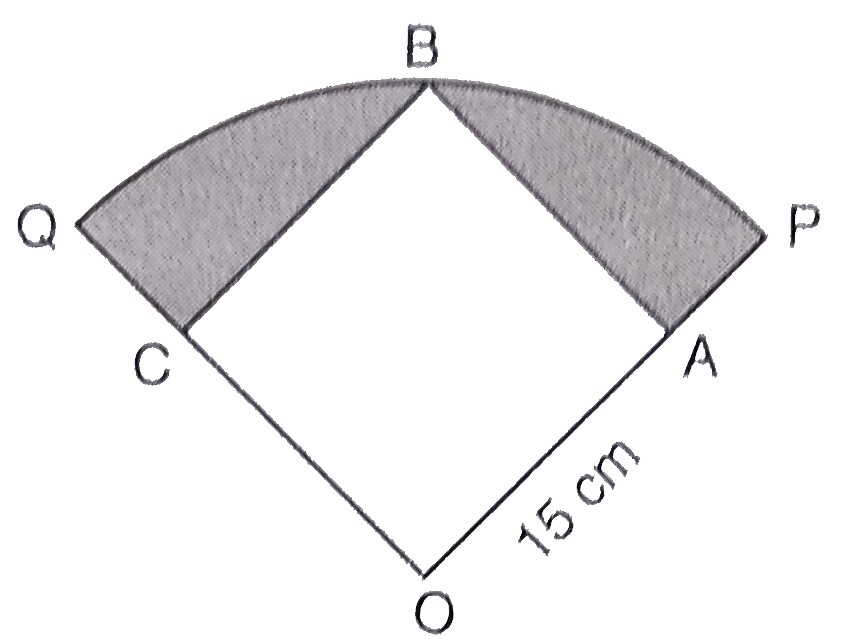"
">

# In the below figure, a square $O A B C$ is inscribed in a quadrant $O P B Q$. If $O A=15 \mathrm{~cm}$, find the area of shaded region (use $\pi=3.14)." Given: A square $O A B C$ is inscribed in a quadrant $O P B Q$ of a circle. $O A=15 \mathrm{~cm}$. To do: We have to find the area of the shaded region. Solution: From the figure,$OABC$is a square.$OA = 15\ cm$Join$OB$, This implies, Diagonal of the square$\mathrm{OB}=\sqrt{2} \times \mathrm{OA}=\sqrt{2} \times 15 \mathrm{~cm}=15 \sqrt{2} \mathrm{~cm}$Radius of the quadrant$=15 \sqrt{2} \mathrm{~cm}$Therefore, Area of the shaded region$=$Area of quadrant$-$Area of square$=\frac{1}{4} \pi r^{2}-(\mathrm{OA})^{2}=\frac{1}{4} \times \frac{22}{7} \times(15 \sqrt{2})^{2}-(15)^{2}=\frac{11}{14} \times 225 \times 2-225=225(\frac{11}{7}-1)=225 \times \frac{11-7}{7}=225\times \frac{4}{7}=128.25 \mathrm{~cm}^{2}$The area of the shaded region is$128.25\ cm^2\$.

Updated on: 10-Oct-2022

30 Views